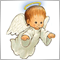# How to calculate the max lot size48

Hi friends,

a small issue that maybe is really simple but i don't find the correct loop to achieve it : I need the function to calculate from a pool of orders Buy with same MagicIndex the maximum Lot Size.

Example :

Run the function and the result is 1.00.... i continue to have the result of 0.01... thanks in advance for your kind help!Moderator
2042448

Keith Watford:

My code is wrong but i try to explain :

Inside the void OnTick i will call the result of the function :

```double calcsize(int MagicIndex)
{
double lotsize= 0.0;

for(int i=OrdersTotal()-1;i>=0;i--){

if(!OrderSelect(i, SELECT_BY_POS)) continue;

if(OrderMagicNumber() != MagicIndex || OrderSymbol() != Symbol()) continue;

lotsize= MathMax(OrderLots(),OrderLots()); //Here is totally wrong but i don't know how to improve...

}

return (lotsize);
}```3025

Onailuig80:

double calcsize(int MagicIndex)

{

double lotsize= 0.0;

for(int i=OrdersTotal()-1;i>=0;i--){

if(!OrderSelect(i, SELECT_BY_POS)) continue;

if(OrderMagicNumber() != MagicIndex || OrderSymbol() != Symbol()) continue;

lotsize= MathMax(OrderLots(),OrderLots()); //Here is totally wrong but i don't know how to improve...

}

return (lotsize);

}

You don't want to calculate but find out the maximum value of Lot size from a pool. Am I right? If so you can try this line instead of yours:

`   lotsize= MathMax(lotsize,OrderLots());`48

Petr Nosek:

You don't want to calculate but find out the maximum value of Lot size from a pool. Am I right? If so you can try this line instead of yours:

It's perferct :-) Thank youuuuuuu